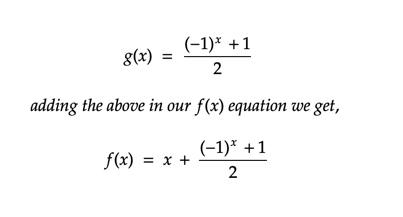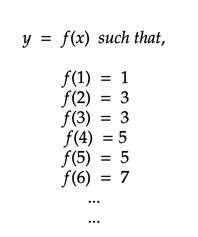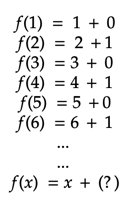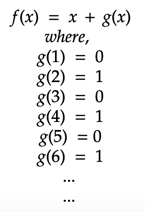# Function to get the preceding odd number (y) for any given number (x)

For any function,We want, for every value of x, return the corresponding odd number. If x is odd then return it as it is, else return the next number. This can easily be done using a computer program by checking if the number is odd or even. But if you had to find a general formula we would need the help of some basic maths.

Let’s decompose our above function and see what we can extract.We just need to find a general formula for the second digit on the RHS which is alternating between 0 and 1. Our function now looks like:The series 0,1,0,1.. can be easily represented by the below formula: